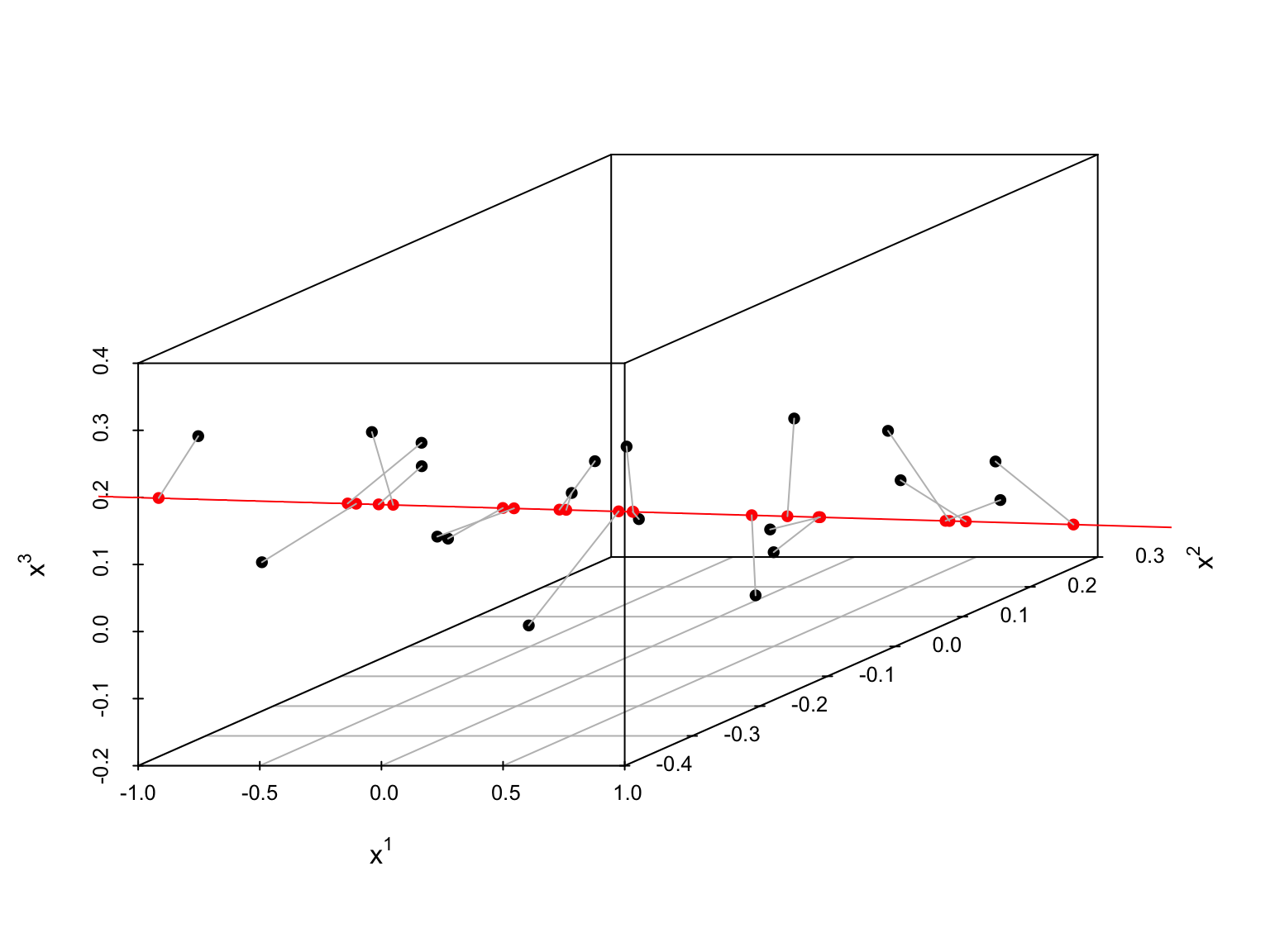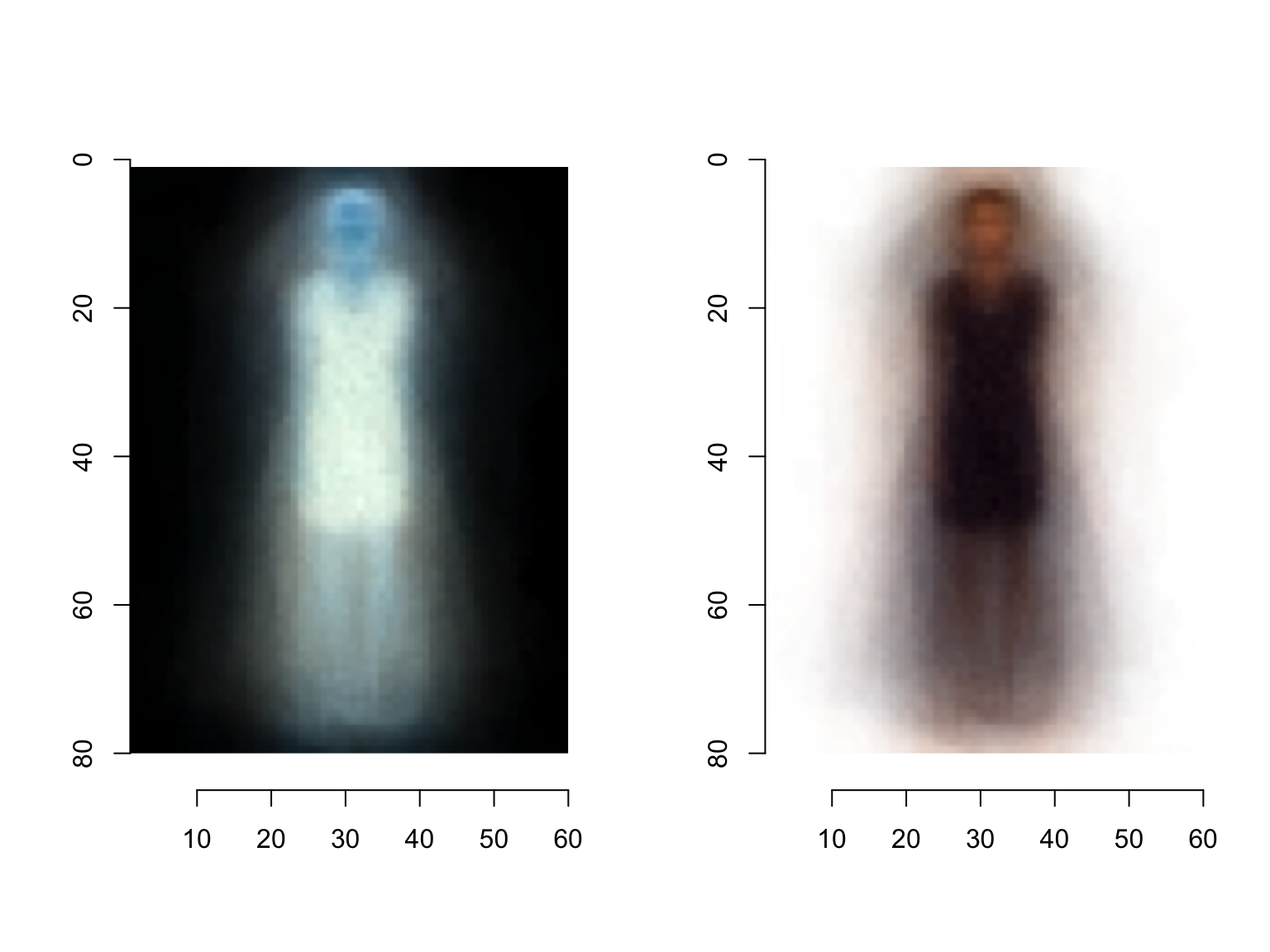# Factor Analysis

16 September 2019

$\newcommand{\X}{\mathbf{x}} \newcommand{\w}{\mathbf{w}} \newcommand{\V}{\mathbf{v}} \newcommand{\S}{\mathbf{s}} \newcommand{\Expect}{\mathbb{E}\left[ #1 \right]} \newcommand{\Var}{\mathrm{Var}\left[ #1 \right]} \newcommand{\SampleVar}{\widehat{\mathrm{Var}}\left[ #1 \right]} \newcommand{\Cov}{\mathrm{Cov}\left[ #1 \right]} \DeclareMathOperator{\tr}{tr} \DeclareMathOperator*{\argmin}{argmin} \newcommand{\FactorLoadings}{\mathbf{\Gamma}} \newcommand{\Uniquenesses}{\mathbf{\psi}}$

# Recap

• Start with $$n$$ items in a data base ($$n$$ big)
• Represent items as $$p$$-dimensional vectors of features ($$p$$ too big for comfort), data is now $$\X$$, dimension $$[n \times p]$$
• Principal components analysis:
• Find the best $$q$$-dimensional linear approximation to the data
• Equivalent to finding $$q$$ directions of maximum variance through the data
• Equivalent to finding top $$q$$ eigenvalues and eigenvectors of $$\frac{1}{n}\X^T \X =$$ sample variance matrix of the data
• New features = PC scores = projections on to the eigenvectors
• Variances along those directions = eigenvalues

# PCA is not a model

• PCA says nothing about what the data should look like
• PCA makes no predictions new data (or old data!)
• PCA just finds a linear approximation to these data
• What would be a PCA-like model?

# This is where factor analysis comes in

Remember PCA: $\S = \X \w$ and $\X = \S \w^T$

(because $$\w^T = \w^{-1}$$)

If we use only $$q$$ PCs, then $\S_q = \X \w_q$ but $\X \neq \S_q \w_q^T$

• Usual approach in statistics when the equations don’t hold: the error is random noise

# The factor model

$$\vec{X}$$ is $$p$$-dimensional, manifest, unhidden or observable

$$\vec{F}$$ is $$q$$-dimensional, $$q < p$$ but latent or hidden or unobserved

The model: $\begin{eqnarray*} \vec{X} & = & \FactorLoadings \vec{F} + \vec{\epsilon}\\ (\text{observables}) & = & (\text{factor loadings}) (\text{factor scores}) + (\text{noise}) \end{eqnarray*}$

# The factor model

$\vec{X} = \FactorLoadings \vec{F} + \vec{\epsilon}$

• $$\FactorLoadings =$$ a $$[p \times q]$$ matrix of factor loadings
• Analogous to $$\w_q$$ in PCA but without the orthonormal restrictions (some people also write $$\w$$ for the loadings)
• Analogous to $$\beta$$ in a linear regression
• Assumption: $$\vec{\epsilon}$$ is uncorrelated with $$\vec{F}$$ and has $$\Expect{\vec{\epsilon}} = 0$$
• $$p$$-dimensional vector (unlike the scalar noise in linear regression)
• Assumption: $$\Var{\vec{\epsilon}} \equiv \Uniquenesses$$ is diagonal (i.e., no correlation across dimensions of the noise)
• Sometimes called the uniquenesses or the unique variance components
• Analogous to $$\sigma^2$$ in a linear regression
• Some people write it $$\mathbf{\Sigma}$$, others use that for $$\Var{\vec{X}}$$
• Means: all correlation between observables comes from the factors
• Not really an assumption: $$\Var{\vec{F}} = \mathbf{I}$$
• Not an assumption because we could always de-correlate, as in homework 2
• Assumption: $$\vec{\epsilon}$$ is uncorrelated across units
• As we assume in linear regression…

# The factor model: summary

$\begin{eqnarray} \vec{X} & = & \FactorLoadings \vec{F} + \vec{\epsilon}\\ \Cov{\vec{F}, \vec{\epsilon}} & = & \mathbf{0}\\ \Var{\vec{\epsilon}} & \equiv & \Uniquenesses, ~ \text{diagonal} \Expect{\vec{\epsilon}} & = & \vec{0}\\ \Var{\vec{F}} & = & \mathbf{I} \end{eqnarray}$

# Some consequences of the assumptions

$\begin{eqnarray} \vec{X} & = & \FactorLoadings \vec{F} + \vec{\epsilon}\\ \Expect{\vec{X}} & = & \FactorLoadings \Expect{\vec{F}} \end{eqnarray}$
• Typically: center all variables so we can take $$\Expect{\vec{F}} = 0$$

# Some consequences of the assumptions

$\begin{eqnarray} \vec{X} & = & \FactorLoadings \vec{F} + \vec{\epsilon}\\ \Var{\vec{X}} & = & \FactorLoadings \Var{\vec{F}} \FactorLoadings^T + \Var{\vec{\epsilon}}\\ & = & \FactorLoadings \FactorLoadings^T + \Uniquenesses \end{eqnarray}$
• $$\FactorLoadings$$ is $$p\times q$$ so this is low-rank-plus-diagonal
• or low-rank-plus-noise
• Contrast with PCA: that approximates the variance matrix as purely low-rank

# Some consequences of the assumptions

$\begin{eqnarray} \vec{X} & = & \FactorLoadings \vec{F} + \vec{\epsilon}\\ \Var{\vec{X}} & = & \FactorLoadings \FactorLoadings^T + \Uniquenesses\\ \Cov{X_i, X_j} & = & \text{what?} \end{eqnarray}$

# Geometry

• As $$\vec{F}$$ varies over $$q$$ dimensions, $$\w \vec{F}$$ sweeps out a $$q$$-dimensional subspace in $$p$$-dimensional space

• Then $$\vec{\epsilon}$$ perturbs out of this subspace

• If $$\Var{\vec{\epsilon}} = \mathbf{0}$$ then we’d be exactly in the $$q$$-dimensional space, and we’d expect correspondence between factors and principal components
• (Modulo the rotation problem, to be discussed)
• If the noise isn’t zero, factors $$\neq$$ PCs
• In extremes: the largest direction of variation could come from a big entry in $$\Uniquenesses$$, not from the linear structure at all

# Geometry# How do we estimate?

$\vec{X} = \FactorLoadings \vec{F} + \vec{\epsilon}$

Can’t regress $$\vec{X}$$ on $$\vec{F}$$ because we never see $$\vec{F}$$

# Suppose we knew$$\Uniquenesses$$

• we’d say $\begin{eqnarray} \Var{\vec{X}} & = & \FactorLoadings\FactorLoadings^T + \Uniquenesses\\ \Var{\vec{X}} - \Uniquenesses & = & \FactorLoadings\FactorLoadings^T \end{eqnarray}$
• LHS is $$\Var{\FactorLoadings\vec{F}}$$ so we know it’s symmetric and non-negative-definite
• $$\therefore$$ We can eigendecompose LHS as $\begin{eqnarray} \Var{\vec{X}} - \Uniquenesses & = &\mathbf{v} \mathbf{\lambda} \mathbf{v}^T\\ & = & (\mathbf{v} \mathbf{\lambda}^{1/2}) (\mathbf{v} \mathbf{\lambda}^{1/2})^T \end{eqnarray}$
• $$\mathbf{\lambda} =$$ diagonal matrix of eigenvalues, only $$q$$ of which are non-zero
• Set $$\FactorLoadings = \mathbf{v} \mathbf{\lambda}^{1/2}$$ and everything’s consistent

# Suppose we knew $$\FactorLoadings$$

then we’d say $\begin{eqnarray} \Var{\vec{X}} & = & \FactorLoadings\FactorLoadings^T + \Uniquenesses\\ \Var{\vec{X}} - \FactorLoadings\FactorLoadings^T & = & \Uniquenesses \end{eqnarray}$

# “One person’s vicious circle is another’s iterative approximation”:

• Start with a guess about $$\Uniquenesses$$
• Suitable guess: regress each observable on the others, residual variance is $$\Uniquenesses_{ii}$$
• Until the estimates converge:
• Use $$\Uniquenesses$$ to find $$\FactorLoadings$$ (by eigen-magic)
• Use $$\FactorLoadings$$ to find $$\Uniquenesses$$ (by subtraction)
• Once we have the loadings (and uniquenesses), we can estimate the scores
small.height <- 80
small.width <- 60
source("../../hw/03/eigendresses.R")
dress.images <- image.directory.df(path = "../../hw/03/amazon-dress-jpgs/",
pattern = "*.jpg", width = small.width, height = small.height)
library("cate")  # For high-dimensional (p > n) factor models
# function is a little fussy and needs its input to be a matrix rather than
# a data frame Also, it has a bunch of estimation methods, but the default
# one doesn't work nicely when some observables have zero variance (here,
# white pixels at the edges of every image --- use something a little more
# robust)
dresses.fa.1 <- factor.analysis(as.matrix(dress.images), r = 1, method = "pc")
summary(dresses.fa.1)  # Factor loadings, factor scores, uniqunesses
##       Length Class  Mode
## Gamma 14400  -none- numeric
## Z       205  -none- numeric
## Sigma 14400  -none- numeric
par(mfrow = c(1, 2))
plot(vector.to.image(dresses.fa.1$Gamma, height = small.height, width = small.width)) plot(vector.to.image(-dresses.fa.1$Gamma, height = small.height, width = small.width))par(mfrow = c(1, 1))
dresses.fa.5 <- factor.analysis(as.matrix(dress.images), r = 5, method = "pc")
summary(dresses.fa.5)
##       Length Class  Mode
## Gamma 72000  -none- numeric
## Z      1025  -none- numeric
## Sigma 14400  -none- numeric